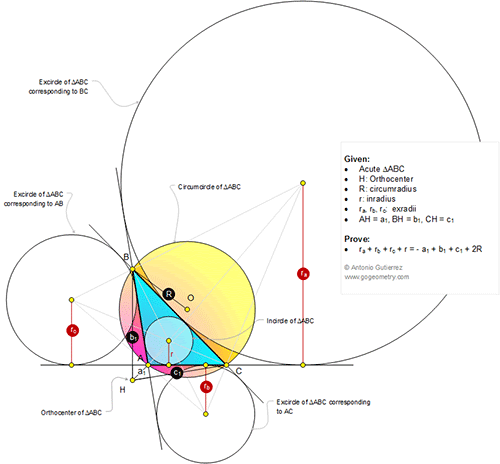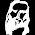## Sunday, December 21, 2014

Level: Mathematics Education, High School, Honors Geometry, College.

Click the diagram below to enlarge it.#### 1 comment:

1.r = 4R sin(A/2) sin(B/2) sin(C/2)
r₁ = 4R sin(A/2) cos(B/2) cos(C/2)
r₂ = 4R cos(A/2) sin(B/2) cos(C/2)
r₃ = 4R cos(A/2) cos(B/2) sin(C/2)

a₁ = −2R cosA
b₁ = 2R cosB
c₁ = 2R cosC

r₁ + r₂ + r₃ − r = 4R
r₁ + r₂ + r₃ + r = 4R + 2r

−a₁ + b₁ + c₁ + 2R = 2R (cosA + cosB + cosC + 1)
= 2R (2 + 4 sin(A/2) sin(B/2) sin(C/2))
= 4R + 2r

Hence, r₁ + r₂ + r₃ + r = −a₁ + b₁ + c₁ + 2R.